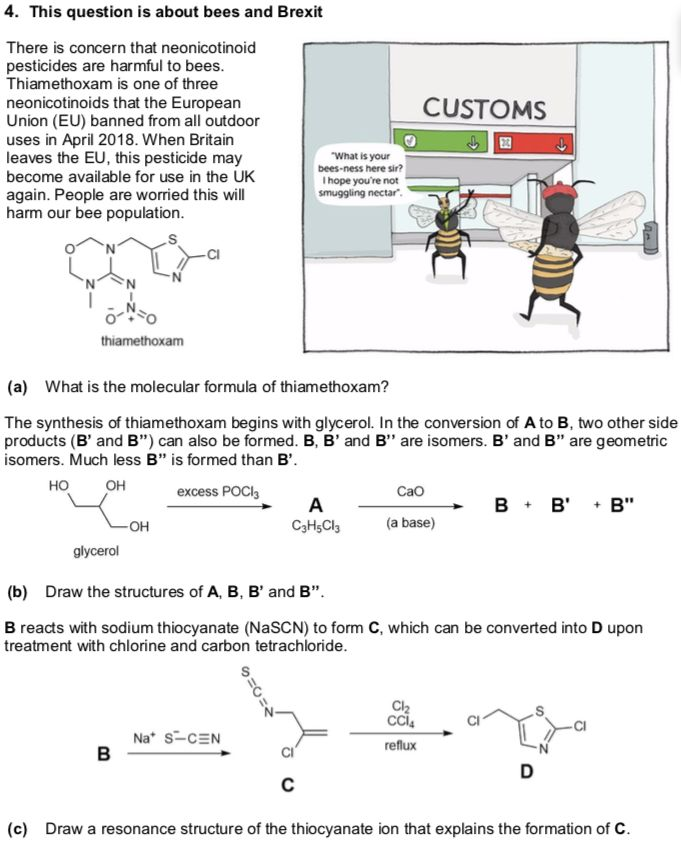# 自动化文件处理

· 阅读需 7 分钟

——陈思达

/docs/science/cavalieri.md
export const Figure = ({children, src}) => (    <div style={{textAlign: 'center'}}>        <img src={src} />        <p style={{color: 'gray', fontSize: 'small'}}>{children}</p>    </div>);...<Figure src="https://mmbiz.qpic.cn/mmbiz_png/JGibibkelET68EfhySWuOboVia7FJX8ehwIAicTz2be2JDN7HIibwibjrpYPP1bTCr1TrjDicauU0P6BLCgFIibZK42GCQ/640?wx_fmt=png&tp=webp&wxfrom=5&wx_lazy=1&wx_co=1"></Figure>1. 按照 URL 下载所有图片，再把它们放在对应的文件夹中（虽然所有东西都是由脚本管理的，但我还是想要保留一些结构化的东西）；
2. 把每个图片的引用改为本地 URL。

## 下载图片​

wget --output-document="correct/path/file.png" 'https://mmbiz.qpic.cn/mmbiz_png/JGibibkelET68EfhySWuOboVia7FJX8ehwIAicTz2be2JDN7HIibwibjrpYPP1bTCr1TrjDicauU0P6BLCgFIibZK42GCQ/640?wx_fmt=png&tp=webp&wxfrom=5&wx_lazy=1&wx_co=1'

1. 我如何生成 correct/path/file.png
2. 我如何定位所有的 URL？

FILE[] files = enumerateFilesUnderPath("./docs/")for file in files:    imagePath = "./static/img/" + removeExtension(file.path)    makeDirectory(imagePath)    string[] links = file.findInFile(/"(?<=<Figure src=\").*?(?=\">)"/g)    for link in links:        imageName = makeSomeMeaningfulName(link)        downloadImage(link, imageName, imagePath)

doc_list=( $(find ./docs -mindepth 2) ) 接下来，我们遍历 doc_list，它包含了每个文件的路径。语法很奇怪，长这样：${doc_list[@]}，而不是直觉所告诉我们的 ${doc_list}（也就是引用 doc_list 变量本身），而只要用过 JS 或者 Python 的 for-each 循环，就更容易想到后者。 doc_list=($(find ./docs -mindepth 2) )for doc in "${doc_list[@]}"do # TODOdone 现在有了 doc 的路径，我们需要在 static 文件夹下生成对应的资源文件夹。doc 路径大概长这样：docs/Science/cavalieri.md。为了把扩展名 .md 修掉，我们要用 "${doc%.*} 这个语法，再在前面添加路径 ./static/img/，就得到了正确的路径 ./static/img/docs/Science/cavalieri，把所有图片放在里面。创建文件夹用的命令是 mkdir

doc_list=( $(find ./docs -mindepth 2) )for doc in "${doc_list[@]}"do    mkdir -p "./static/img/""${doc%.*}" # TODOdone 运行一遍这个脚本，我们就能得到正确的文件树，但没有任何内容。 接下来，我们要从文件中提取出 URL。要用正则在文件中搜索，可以用命令 grep。所有的 URL 都被包含在这样的格式中：<Figure src="...">，所以最自然的做法就是用正则前瞻和后瞻。不幸的是，苹果系统中的 grep 不支持 Perl，所以为了用 -p 选项，我需要安装 grep，它提供了 GNU 风格的 ggrep。现在我们就能抓取出所有的链接了。 doc_list=($(find ./docs -mindepth 2) )for doc in "${doc_list[@]}"do mkdir -p "./static/img/""${doc%.*}"    links=( $(ggrep -o -P "(?<=<Figure src=\").*?(?=\">)" "$doc") )    # TODOdone

doc_list=( $(find ./docs -mindepth 2) )for doc in "${doc_list[@]}"do    mkdir -p "./static/img/""${doc%.*}" links=($(ggrep -o -P "(?<=<Figure src=\").*?(?=\">)" "$doc") ) for link in "${links[@]}"    do        name=$(echo "$link" | ggrep -o -P "(?<=(jpg|png)/).*(?=/640)")        ext=$(echo "$link" | ggrep -o -P "(?<=wx_fmt=).*")        # Almost there!    donedone

doc_list=( $(find ./docs -mindepth 2) )for doc in "${doc_list[@]}"do    mkdir -p "./static/img/""${doc%.*}" links=($(ggrep -o -P "(?<=<Figure src=\").*?(?=\">)" "$doc") ) for link in "${links[@]}"    do        name=$(echo "$link" | ggrep -o -P "(?<=(jpg|png)/).*(?=/640)")        ext=$(echo "$link" | ggrep -o -P "(?<=wx_fmt=).*")        wget --output-document="./static/img/""${doc%.*}""/"$name"."$ext "$link"    donedone## 更改 URL​

/docs/science/cavalieri.md
<Figure src="https://mmbiz.qpic.cn/mmbiz_png/JGibibkelET68EfhySWuOboVia7FJX8ehwIAicTz2be2JDN7HIibwibjrpYPP1bTCr1TrjDicauU0P6BLCgFIibZK42GCQ/640?wx_fmt=png&tp=webp&wxfrom=5&wx_lazy=1&wx_co=1"></Figure>

/docs/science/cavalieri.md
<Figure src="/zh-Hans/img/docs/Science/cavalieri/JGibibkelET68EfhySWuOboVia7FJX8ehwIAicTz2be2JDN7HIibwibjrpYPP1bTCr1TrjDicauU0P6BLCgFIibZK42GCQ.png"></Figure>